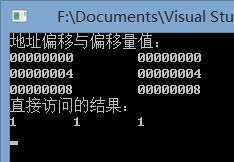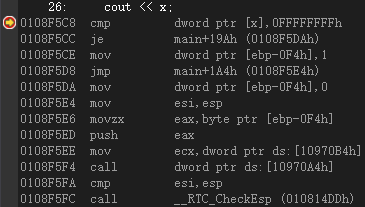# C++：偏移类型

Warning: WP_Syntax::substituteToken(): Argument #1 (\$match) must be passed by reference, value given in /www/wwwroot/fawdlstty.com/wp-content/plugins/wp-syntax/wp-syntax.php on line 383

Warning: WP_Syntax::substituteToken(): Argument #1 (\$match) must be passed by reference, value given in /www/wwwroot/fawdlstty.com/wp-content/plugins/wp-syntax/wp-syntax.php on line 383

```1 2 3 4 5 6 7 8 9 10 11 12 13 14 15 16 17 18 19 20 21 22 23 24 25 26 27 #include <iostream> using namespace std;   class A { public: int a, b, c; A () : a (3), b (5), c (7) {} };   int main (int argc, char* argv[]) { int (A::* x) = &A::a; int A::* y = &A::b; using TZ = int (A::*); TZ z = &A::c;   A *p = (A*)nullptr;   cout << "地址偏移与偏移量值：" << endl; cout << &(p->a) << '\t' << &(p->*x) << endl; cout << &(p->b) << '\t' << &(p->*y) << endl; cout << &(p->c) << '\t' << &(p->*z) << endl;   cout << "直接访问的结果：" << endl; cout << x << '\t' << y << '\t' << z << endl;   return 0; }``````1 2 __asm { mov x, 0ffffffffh } cout << x;```

## 发布者